# Texas Go Math Grade 3 Lesson 19.1 Answer Key Frequency Tables

Refer to our Texas Go Math Grade 3 Answer Key Pdf to score good marks in the exams. Test yourself by practicing the problems from Texas Go Math Grade 3 Lesson 19.1 Answer Key Financial Decisions.

## Texas Go Math Grade 3 Lesson 19.1 Answer Key Frequency Tables

Unlock the Problem

The students in Alicia’s class voted for their favorite ice cream flavor. They organized the data in this tally table. How many more students chose chocolate than strawberry?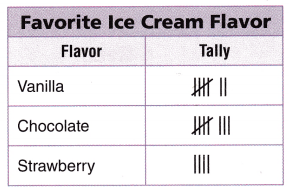Another way to show the data is in a frequency table. A frequency table uses numbers to record data.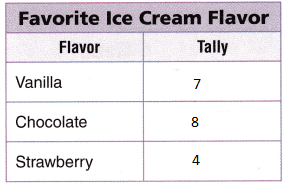Explanation:
The number of students choose chocolate is 8
The number of students choose strawberry is 4
8 – 4 = 4
4 more students chose chocolate than strawberry

What do I need to find?
How many more students chose ________ than __________ ice cream as their favorite?

What information am I given?
the data about favorite __________ in the tally table

What do I need to find?
How many more students chose chocolate than Strawberry ice cream as their favorite?

What information am I given?
the data about favorite ice cream in the tally table

Plan

What is my plan or strategy?
I will count the __________. Then I will put the numbers in a frequency table and compare the number of students

who chose ___________ to the number of students who chose ___________.
Explanation:

What is my plan or strategy?
I will count the number. Then I will put the numbers in a frequency table and compare the number of students

who chose chocolate to the number of students who chose strawberry.

SolveCount the tally marks. Record _________ for vanilla. Write the other flavors and record the number of tally marks.

To compare the number of students who chose strawberry and the number of students who chose chocolate, subtract.
__________ – ___________ = _________

So, _________ more students chose chocolate as their favorite flavor.Count the tally marks. Record points for vanilla. Write the other flavors and record the number of tally marks.

To compare the number of students who chose strawberry and the number of students who chose chocolate, subtract.
8 – 4 = 4

So, 4 more students chose chocolate as their favorite flavor.

Try Another Problem

Two classes in Carter’s school grew bean plants for a science project. The heights of the plants after six weeks are shown in the tally table. The plants were measured to the nearest inch. How many fewer bean plants were 9 inches tall than 7 inches and 8 inches combined?What do I need to find?

What information am I given?

Plan

What is my plan or strategy?

Solve

Record the steps you used to solve the problem.Suppose the number of 3-inch plants was half the number of 8-inch plants. How many 3-inch bean plants were there?
Explanation:
Read: How many fewer bean plants were 9 inches tall than 7 inches and 8 inches combined?
Plan: Count how many 9 inches bean plants are there and 7 inch bean plants and 8 inch bean plants
Then added 7 and 8 inch bean plants
7 inch bean plants are 9
8 inch bean plants are 8, 9 + 8 = 17
9 inch bean plants are 12
17 – 12 = 5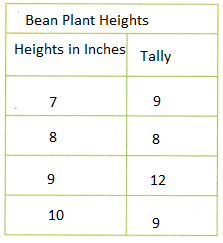The number of 3-inch plants was half the number of 8-inch plants.
8 inch bean plants are 8 so half is4
3-inch bean plants were 4

Math Talk
Mathematical Processes

Explain another strategy you could use to solve the problem.
Explanation:
Just count the lines
subtract number of 8 inch + 7 inch and 9 inch
so, the answer is 17 – 12 = 5

Share and Show

Use the Shoe Lengths table for 1-4.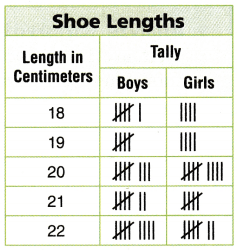Question 1.
The students in three third-grade classes recorded the lengths of their shoes to the nearest centimeter. The data are in the tally table. Count the tally marks and put the data in a frequency table. How many more shoes were 18 or 22 centimeters long combined than 20 centimeters long?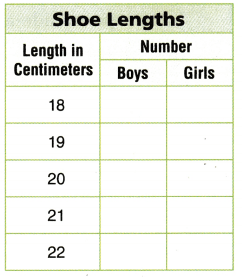To find the number of shoes that were 18 or 22 centimeters long, add
6 + _______+ _______ + _______ = _______.
To find the number of shoes that were 20 centimeters long, add _______ + _______ = _______.

To find the difference between the shoes that were 18 or 22 centimeters long and the shoes that were 20 centimeters long, subtract the sums.
_______ – _______ = _______
So, _______ more shoes were 18 or 22 centimeters long than 20 centimeters long.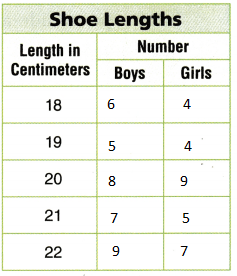To find the number of shoes that were 18 or 22 centimeters long, add
6 + 4+ 9 + 7 = 26.
To find the number of shoes that were 20 centimeters long, add 8 + 9 = 17.

To find the difference between the shoes that were 18 or 22 centimeters long and the shoes that were 20 centimeters long, subtract the sums.
26 – 17 = 9
So, 9 more shoes were 18 or 22 centimeters long than 20 centimeters long.

Question 2.
How many fewer boys’ shoes were 19 cm long than 22 cm long?
Explanation:
4 fewer boys’ shoes were 19 cm long than 22 cm long
19cm long is 5
22cm long is 9
9-5 = 4

Problem Solving

Question 3.
H.O.T. What if the length of 5 more boys’ shoes measured 21 centimeters? Explain how the table would change.Explanation:
if the length of 5 more boys’ shoes measured 21 centimeters.
the above table shows how it changed
shoes measured 21 centimeters. is 7
7 + 5 = 12

Question 4.
H.O.T. Multi-Step How many fewer girls’ shoes than boys’ shoes were measured?
Explanation:
The number of boys shoes are 12 and the number of girl shoes are 5
12 – 5 = 7

Fill in the bubble for the correct answer choice. Use Favorite Outdoor Game frequency table for 5-7.Question 5.
Analyze Raj asked his classmates to choose their favorite outdoor game. His results are shown in the frequency table at the right. How many more students choose hide-and-seek than scavenger hunt?
(A) 8
(B) 20
(C) 14
(D) 9
Explanation:
Raj asked his classmates to choose their favorite outdoor game.
His results are shown in the frequency table at the right.
8 more students choose hide-and-seek than scavenger hunt
students choose hide-and-seek is 14
students choose  scavenger hunt is 6
14 – 6 = 8

Question 6.
Multi-Step How many students in all chose tag, jump rope, or hide-and-seek?
(A) 31
(B) 39
(C) 25
(D) 23
Explanation:
39 students in all chose tag, jump rope, or hide-and-seek
14 + 16 + 9 = 39

Question 7.
Multi-Step How many more students chose tag than jump rope and scavenger hunt combined?
(A) 2
(B) 1
(C) 15
(D) 7
Explanation:
1 more  than jump rope and scavenger hunt combined
students chose tag is 16
jump rope and scavenger hunt is 15
16 – 15 = 1

Texas Test Prep

Question 8.
Representations Jade made a tally table to record how many people have different types of pets. Her table shows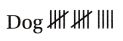How many people have dogs?
(A) 9
(B) 4
(C) 14
(D) 15
Explanation:
the number of lines representing the dog is 14
14 people have dogs

### Texas Go Math Grade 3 Lesson 19.1 Homework and Practice Answer Key

Problem Solving

Use the Favorite Pet table for 1-7.Question 1.
The students in third grade were asked to choose their favorite pet. The data are in the tally table. Count the tally marks and put the data in a frequency table.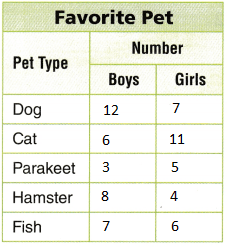Explanation:
The students in third grade were asked to choose their favorite pet.
The data are in the tally table.
Counted the tally marks and kept the data in a frequency table.

Question 2.
How many more boys than girls chose dog as the favorite pet
Answer: 12 – 7 = 5
Explanation:
5  more boys than girls chose dog as the favorite pet

Question 3.
How many students chose cat as the favorite pet?
17 students chose cat as the favorite pet

Question 4.
How many more students chose fish than parakeets?
Explanation:
5 more students chose fish than parakeets
number of fishes is 13
number of parakeets is 8
13 – 8 = 5

Question 5.
Which pet got the least number of votes?
Explanation:
parakeets pet got the least number of votes that is 8

Question 6.
How many more students chose cat or dog combined than parakeet or hamster combined?
Explanation:
16 more students chose cat or dog combined than parakeet or hamster combined
cat or dog combined is 12 + 7 + 6 + 11 = 36
parakeet or hamster combined is 3 + 5 + 8 + 4 = 20
36 – 20 = 16

Question 7.
Did more boys or girls choose a  favorite pet? Explain?
Explanation:
boys chosen the pet are 12 + 6 + 3 + 7 = 36
girls chosen the pet are 7 + 11 + 5 + 4 + 6 =33
3 more boys than girls

Lesson Check

Fill in the bubble completely to show your answer. Use the Favorite Book table for 8-10.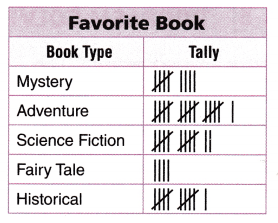Question 8.
Ernie asked the third-grade classes in his school to choose their favorite type of book. How many more students chose adventure books than fairy tale books?
(A) 12
(B) 16
(C) 4
(D) 20
Explanation:
Fairy tale book is 4
16 – 4 = 12

Question 9.
How many students in all chose mystery or science fiction?
(A) 9
(B) 12
(C) 21
(D) 3
Explanation:
students chose mystery is 9
students chose science fiction is 12
9 + 12 = 21
21 students in all chose mystery or science fiction

Question 10.
Multi-Step How many more students chose historically and science fiction combined than adventure?
(A) 23
(B) 16
(C) 7
(D) 8
Explanation:
students choose historically is 11
student chose science fiction is 12
11 + 12 = 23
23 – 16 = 7 more students chose historically and science fiction combined than adventure

Use the Books Read During Summer table for 11-13.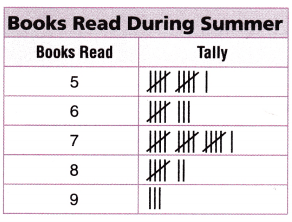Question 11.
Halle recorded in a tally table the number of books that students read during summer vacation. How many more students read 7 books than 5 books during summer vacation?
(A) 2
(B) 5
(C) 12
(D) 27
Explanation:
Halle recorded in a tally table the number of books that students read during summer vacation.
5 more students read 7 books than 5 books during summer vacation
students read 7 books are 16
students read 5 books are 11
16 – 11 = 5

Question 12.
How many students read 8 books during summer vacation?
(A) 15
(B) 4
(C) 16
(D) 7
Explanation:
7 students read 8 books during summer vacation

Question 13.
Multi-Step How many more students read 7 books than 6 and 9 books combined?
(A) 11
(B) 13
(C) 4
(D) 5